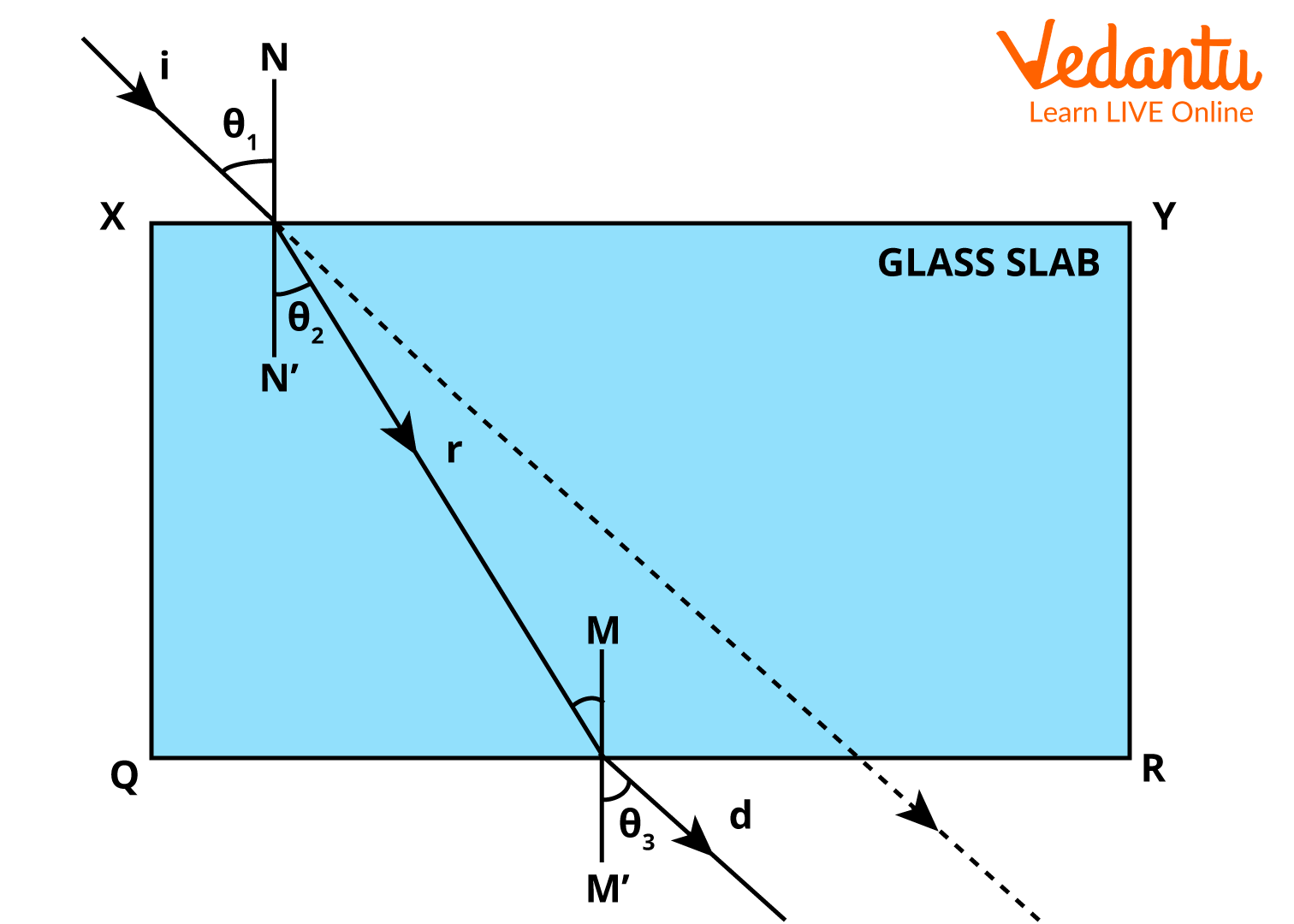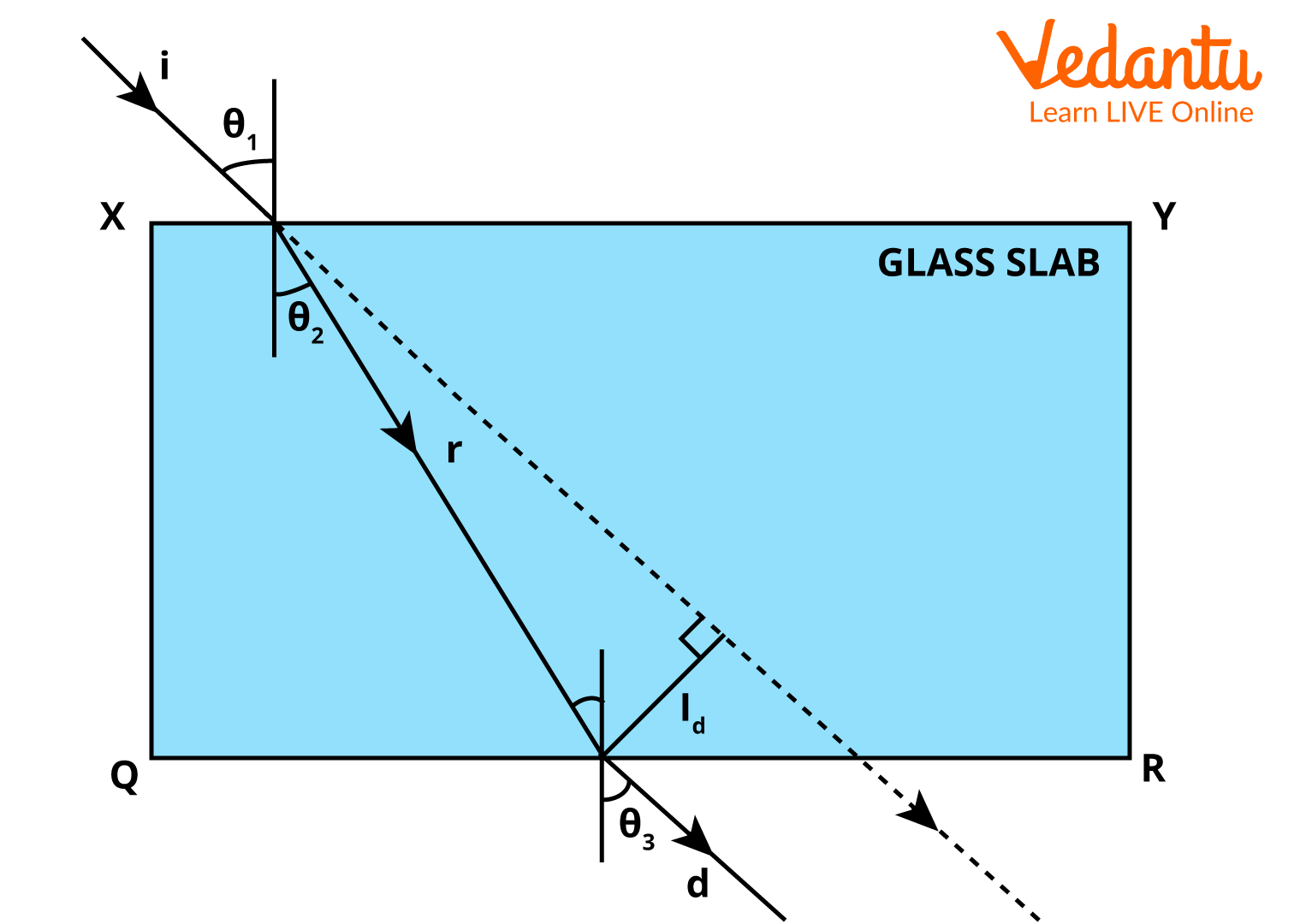Courses
Courses for Kids
Free study material
Free LIVE classes
More

# Refraction of Light Through a Glass Slab for JEELIVE
Join Vedantu’s FREE Mastercalss

## What is Refraction?

When a light wave passes from one medium to another, it gets redirected. That means when it passes through another medium it changes its direction and later when it comes out of that medium, it retains its original direction in a glass slab. This phenomenon is called refraction. The light waves and other waves like sound or water waves also possess this phenomenon of refraction. In essence, this refraction could only be seen when there is a change in the speed of the wave or in the medium in which the wave travels.

Refraction follows a law called Snell’s Law, which states that when there are two media, the ratio of sines of the angle of incidence, say $\theta_1$ and the angle of refraction say $\theta_2$, is equal to the ratio of the velocities of the wave in two media and also equal to the inverse ratio of the refractive indices of those media.

This is represented as shown below.

$\dfrac{\sin \theta_{1}}{\sin \theta_{2}}=\dfrac{v_{1}}{v_{2}}=\dfrac{n_{2}}{n_{1}}$

Here, the angle of incidence is an angle between the ray and the normal at which the ray falls on the surface of a medium. And the angle of refraction is the angle at which the ray gets refracted from the normal after getting refracted into the media.

Let’s know how the refraction of light through a glass slab works out.

## Refraction of Light Through a Glass Slab

Let us draw a diagram where an incident ray of light which is travelling in a vacuum falls on the surface of the glass slab.Refraction Through Glass Slab

As it is obvious from the diagram, the incident ray is falling on the surface of the glass slab, i.e., XY at an angle of incidence $\theta_1$ with the normal. Then the refraction phenomenon takes place and the wave gets deviated from its actual path when it enters the medium of the glass slab. Here, the angle made by the refracted ray with the normal is $\theta_2$ and this deviated ray, d goes away from the normal.

Note: if the ray is incident on the XY surface of the glass slab along the normal making 90 degrees with the surface XY, then there will be no deviation in the ray's path.

After the refraction at the surface XY, the ray passes through and falls on the surface of the glass slab on the other side, i.e., QR. Thus, one more refraction takes place here. Here, the refracted ray from the surface XY becomes the incident ray for the surface QR at an angle of incidence $\theta_2$ and gets refracted. The final ray coming out of the surface of the glass slab, QR, is called the emergent ray with an angle of emergence $\theta_3$. The path of the emergent ray will be surely parallel to the path of the first incident ray.

There is an important law of refraction here to be noticed; at the point of incidence on the surface XY, the incident ray, which is normal to the surface and the refracted ray lie in the same plane. There is the second law of refraction that has been discussed already. That is represented as shown below.

$\dfrac{\sin i}{\sin r}=$ constant

Where i is the angle of incidence and r is the angle of refraction.

Also, this value depends only on the refractive indices of the two media. The Refractive index of a medium is a measure of the reduction in velocity of light in the medium when compared to that of the vacuum. Therefore, Snell's law is described in a mathematical form as

$\dfrac{\sin i}{\sin r}=\dfrac{n_{r}}{n_{i}}$

Here, ni and nr are the refraction indices of the incident and refracted media respectively.

The above equation can be written as

ni sin i = nr sin r

This is called snell’s law and this must be satisfied if refraction wants to happen in any media.

Therefore, we discussed how to explain refraction through a rectangular glass slab with the help of a diagram of refraction of light through a glass slab.

## Lateral Displacement

Let’s take the diagram drawn for refraction through a glass slab to understand the concept of the lateral shift. In the below figure, as we can see, a black line is drawn from the point of incidence and along the path of the incident ray.Lateral Displacement in a Glass Slab

This is the path that would be followed by the light ray if it was travelling in the vacuum alone without entering the medium of a rectangular glass slab. This is the actual path of the incident ray. Now, from the point of emergence, a perpendicular line is drawn to the incident ray, I which is almost parallel to the emergent or deviated ray, d on the surface QR.

The length of the line drawn perpendicularly to the incident ray from the point of emergence is called Lateral Displacement or Later Shift, which is represented by the letter Ld. To be concise, the perpendicular distance between the incident ray and the emergent ray is called Lateral Displacement.

The lateral displacement formula is given by the equation

$l_{d}=t \dfrac{\sin (i-r)}{\cos r}$

Where ld is the lateral shift or displacement.

i, r are the angles of incidence and the refraction, respectively.

t is the thickness of the glass slab acting as a medium.

Therefore, the concept of lateral displacement is explained with the lateral displacement formula. Let’s conclude what we’ve discussed till now.

## Conclusion

In conclusion, we have learnt how to explain refraction through a rectangular glass slab with the help of a diagram and valid formulae. We have to keep in mind that refraction through glass slabs takes place twice, one at the incident point and one at the emergent point. Therefore, in the article, lateral shift is discussed and Snell’s law is also discussed with a proper derivation.

Last updated date: 27th Sep 2023
Total views: 3.3k
Views today: 0.03k

## FAQs on Refraction of Light Through a Glass Slab for JEE

1. What are the two laws of refraction?

There are two laws of refraction which every media must satisfy to have room for the occurrence of the refraction phenomenon. The first one states that,  at the surface of the incidence where two media interact, the incident ray, the refracted ray, and the normal to the surface lie in the same plane. The second law states that the ratio of the sine of the angle of refraction and the sine of the angle of incidence is constant and depends only on the refractive indices of the media. This is also addressed as Snell’s Law of Refraction.

2. What is the difference between refraction through glass slab and prism?

The significant difference between refraction through glass slab and prism is that in a glass slab, the emergent ray from the opposite side of the glass slab will be almost parallel to the actual path of the incident ray. Here, there is no kind of dispersion of rays but just a displacement laterally. But in a prism, the emergent ray will not be parallel to the incident ray instead it gets deviated from its path with an angle. In prism, splitting of light also occurs.